Olympiad Test: Odd One Out - 2

# Olympiad Test: Odd One Out - 2 - Class 7

Test Description

## 10 Questions MCQ Test Science Olympiad Class 7 - Olympiad Test: Odd One Out - 2

Olympiad Test: Odd One Out - 2 for Class 7 2023 is part of Science Olympiad Class 7 preparation. The Olympiad Test: Odd One Out - 2 questions and answers have been prepared according to the Class 7 exam syllabus.The Olympiad Test: Odd One Out - 2 MCQs are made for Class 7 2023 Exam. Find important definitions, questions, notes, meanings, examples, exercises, MCQs and online tests for Olympiad Test: Odd One Out - 2 below.
 1 Crore+ students have signed up on EduRev. Have you?
Olympiad Test: Odd One Out - 2 - Question 1

### Directions: In each problem, out of the five figures marked (1), (2), (3), (4) and (5), four are similar in a certain manner. However, one figure is not like the other four. Q. Choose the figure which is different from the rest.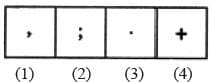Detailed Solution for Olympiad Test: Odd One Out - 2 - Question 1

All others are punctuation marks.

Olympiad Test: Odd One Out - 2 - Question 2

### Directions: In each problem, out of the five figures marked (1), (2), (3), (4) and (5), four are similar in a certain manner. However, one figure is not like the other four. Q. Choose the figure which is different from the rest.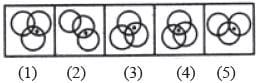Detailed Solution for Olympiad Test: Odd One Out - 2 - Question 2

Only in fig. (4), the dot appears in the region common to all the three circles.

Olympiad Test: Odd One Out - 2 - Question 3

### Directions: In each problem, out of the five figures marked (1), (2), (3), (4) and (5), four are similar in a certain manner. However, one figure is not like the other four. Q. Choose the figure which is different from the rest.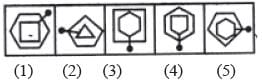Detailed Solution for Olympiad Test: Odd One Out - 2 - Question 3

Only in fig. (4), the pin passes through a vertex of each one of the two elements.

Olympiad Test: Odd One Out - 2 - Question 4

Directions: In each problem, out of the five figures marked (1), (2), (3), (4) and (5), four are similar in a certain manner. However, one figure is not like the other four.

Q. Choose the figure which is different from the rest.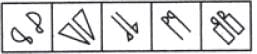Detailed Solution for Olympiad Test: Odd One Out - 2 - Question 4

All other figures contain a geometrical figure along with its mirror image.

Olympiad Test: Odd One Out - 2 - Question 5

Directions: In each problem, out of the five figures marked (1), (2), (3), (4) and (5), four are similar in a certain manner. However, one figure is not like the other four.

Q. Choose the figure which is different from the rest.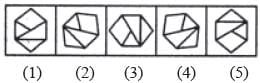Detailed Solution for Olympiad Test: Odd One Out - 2 - Question 5

All other figures can be rotated into each other.

Olympiad Test: Odd One Out - 2 - Question 6

Directions: In each problem, out of the five figures marked (1), (2), (3), (4) and (5), four are similar in a certain manner. However, one figure is not like the other four.

Q. Choose the figure which is different from the rest.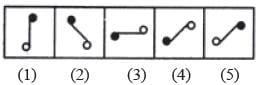Detailed Solution for Olympiad Test: Odd One Out - 2 - Question 6

Figure (c) is different from other figures.

Olympiad Test: Odd One Out - 2 - Question 7

Directions: In each problem, out of the five figures marked (1), (2), (3), (4) and (5), four are similar in a certain manner. However, one figure is not like the other four.

Q. Choose the figure which is different from the rest.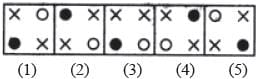Detailed Solution for Olympiad Test: Odd One Out - 2 - Question 7

In each one of the figures except fig. (3), the two crosses (x) appear in the diagonally opposite corners.

Olympiad Test: Odd One Out - 2 - Question 8

Directions: In each problem, out of the five figures marked (1), (2), (3), (4) and (5), four are similar in a certain manner. However, one figure is not like the other four.

Q. Choose the figure which is different from the rest.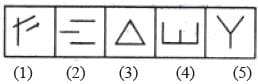Detailed Solution for Olympiad Test: Odd One Out - 2 - Question 8

Figure (4) is formed by four line segments while each one of the other figures is formed by three line segments.

Olympiad Test: Odd One Out - 2 - Question 9

Directions: In each problem, out of the five figures marked (1), (2), (3), (4) and (5), four are similar in a certain manner. However, one figure is not like the other four.

Q. Choose the figure which is different from the rest.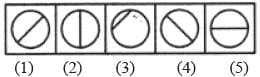Detailed Solution for Olympiad Test: Odd One Out - 2 - Question 9

Only in fig. (3), the line segment is not a diameter of the circle.

Olympiad Test: Odd One Out - 2 - Question 10

Directions: In each problem, out of the five figures marked (1), (2), (3), (4) and (5), four are similar in a certain manner. However, one figure is not like the other four.

Q. Choose the figure which is different from the rest.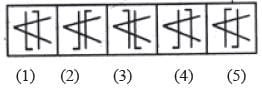Detailed Solution for Olympiad Test: Odd One Out - 2 - Question 10

Only in fig. (4), both the parallel lines are bent in the same direction {i.e. towards the left).(Scan QR code)